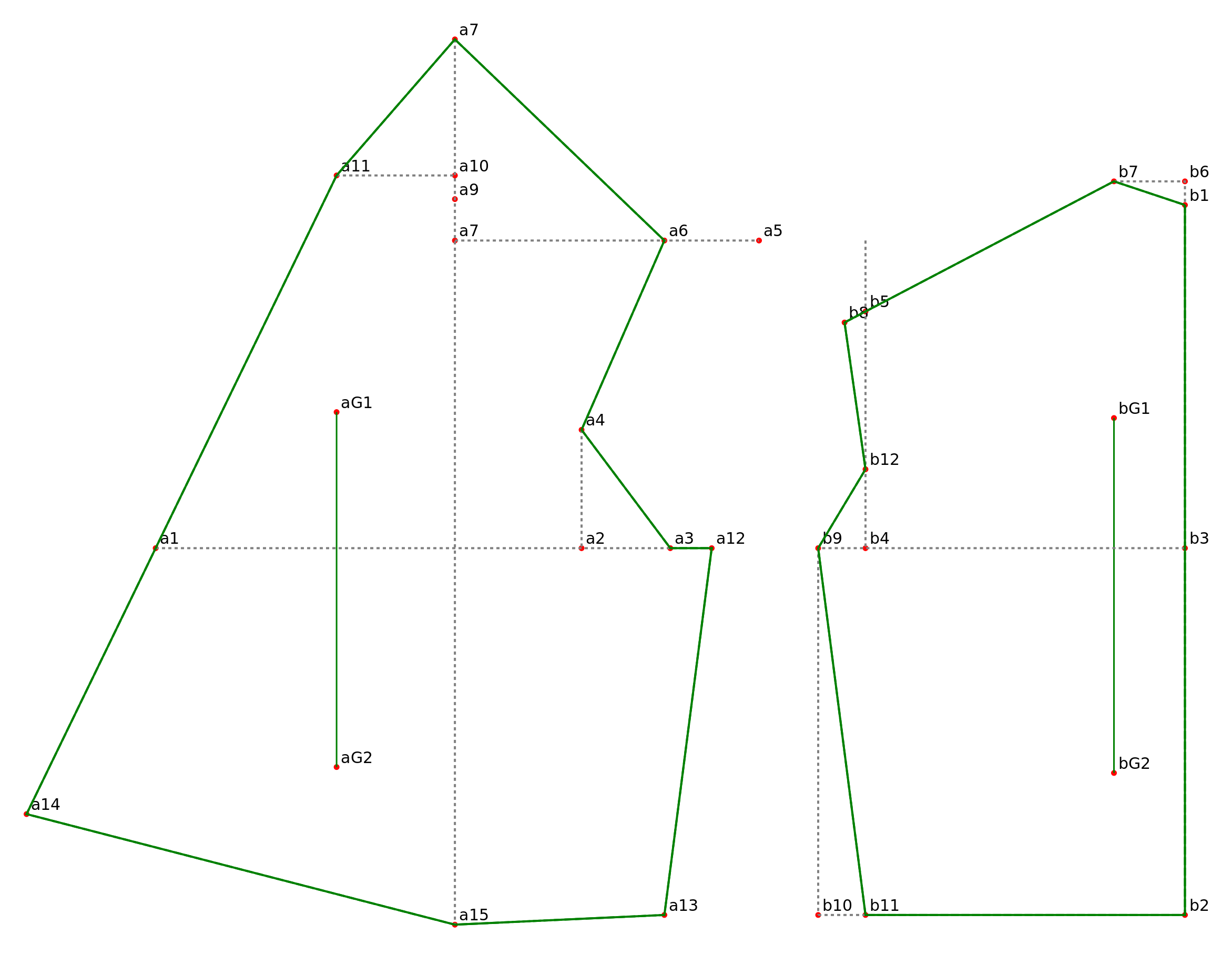# Creating a Pattern as an Inkscape Extension: Part 3

 Continuation of  Part 2 STEP 6: CREATE THE SVG OBJECTS The formulas we’ve written calculate the x,y coordinates for each point. They don’t create the SVG elements that we see on the Inkscape canvas. If we wrap each of these formulas with a function that creates an SVG element, then we calculate the pattern point coordinates and create the SVG element in one step. The functions patternPoint(parent,id,point) and patternPointXY(parent,id,x,y) create small red circles.  The parent is the pattern piece where the pattern point belongs . A is the front pattern piece, B is the back pattern piece. The SVG id should be the same as the python variable name so that there is a one-to-one mapping from the python variables to the SVG elements.  It is the SVG id not the python variable name that is displayed next to each pattern point. b1=patternPointXY(B,’b1′,0,0) b2=patternPoint(B,’b2′,downPoint(b1, front_waist_length)) b3=patternPoint(B,’b3′,upPoint(b2, side)) a1=patternPoint(A,’a1′,leftPoint(b3, bust_circumference/2.0)) b4=patternPoint(B,’b4′,leftPoint(b3, across_back/2.0)) b5=patternPoint(B,’b5′,upPoint(b4, armscye_circumference/3.0)) b6=patternPoint(B,’b6′,upPoint(b1, 0.5*IN)) b7=patternPoint(B,’b7′,leftPoint(b6,1.5*IN)) b8=patternPoint(B,’b8′,intersectLineAtLength(b5,b7, -0.5*IN)) a2=patternPoint(A,’a2′,leftPoint(b4, armscye_circumference/4.0)) a3=patternPoint(A,’a3′,midPoint(a2,b4)) a4=patternPoint(A,’a4′,upPoint(a2.x, 2.5*IN)) a5=patternPoint(A,’a5′,upPoint(b5.x, 1.5*IN)) a6=patternPoint(A,’a6′,leftPoint(a5, 2*IN)) a7=patternPoint(A,’a7′,leftPoint(a5, distance(b7,b8))) a8=patternPointXY(A,’a7′,a7.x, b3.y-upper_front_height) a9=patternPoint(A,’a9′,downPoint(a8, neck_circumference/4.0)) a10=patternPoint(A,’a10′,upPoint(a9, 0.5*IN)) a11=patternPoint(A,’a11′,leftPoint(a10, (neck_circumference/6.0)+0.25*IN )) b9=patternPoint(B,’b9′,leftPoint(b4, 1*IN)) a12=patternPoint(A,’a12′,b9) b10=patternPoint(B,’b10′,downPoint(b9, side)) b11=patternPoint(B ,’b11′,rightPoint(b10, 1*IN)) a13=patternPoint(A,’a13′,leftPoint(b10, 1*IN)) a14=patternPoint(A,’a14′,intersectLineAtLength(a11, a1, front_waist_length)) a15=patternPoint(A,’a15′,downPoint(a8, distance(a8, a14))) b12=patternPoint(B,’b12′,downPoint(b5, (2/3.0)*distance(b5, b4))) curve_a3b12=pointList(a3, b12.c1, b12.c2, b12) b13=patternPoint(B,’b13′,intersectLineCurve(b9,b10,curve_a3b12)) a15=patternPoint(A,’a15′,b13) STEP 7: PUT THE FORMULAS INTO A PYTHON FILE Download  shirt_waist_allington_1.inx, shirt_waist_allington_1.py, and sewing_patterns.py and save to your inkscape user extension directory.  For Linux, this directory is /\$HOME/.config/inkscape/extensions.  For Windows it’s C:\Program Files\Inkscape\extensions. For MAC it’s ~/.config/inkscape/extensions. Open the shirt_waist_allington_1.py file.  All of the measurements are converted to pixels with MEASUREMENT_CONVERSION: #convert measurements front_waist_length=self.options.m_front_waist_length*MEASUREMENT_CONVERSION neck_circumfrence=self.options.m_neck_circumfrence*MEASUREMENT_CONVERSION bust_circumference=self.options.m_bust_circumference*MEASUREMENT_CONVERSION waist_circumference=self.options.m_waist_circumference*MEASUREMENT_CONVERSION armscye_circumference=self.options.m_armscye_circumference*MEASUREMENT_CONVERSION across_back=self.options.m_across_back*MEASUREMENT_CONVERSION side=self.options.m_side*MEASUREMENT_CONVERSION upper_front_height=self.options.m_upper_front_height*MEASUREMENT_CONVERSION All of the formulas have been pasted in the section labeled #pattern points. The formulas for curve_a3b12, b13, and a15 have been commented out. The path for the front bodice starts and ends at a11. We’re checking the point formulas, so there are no curves, only Moveto and Line commands. Here is the command that creates the path string used for the seamline and cuttingline paths for the front bodice A: path_str=formatPath(‘M’,a11,’L’,a14,’L’,a15,’L’,a13,’L’,a12,’L’,a3,’L’,a4,’L’,a6,’L’,a8,’L’,a11) A_seamline=addPath(A,’A_seamline’,path_str,’seamline’) A_cuttingline=addPath(A,’A_cuttingline’,path_str,’cuttingline’) Tau Meta only uses Move ‘M’, Lineto ‘L’ and Curve ‘C’ commands.  There are no relative move ‘m’, lineto ‘l’, curve ‘c’, horizontal ‘h’,  vertical ‘v’, or close ‘z’ commands.After the files are in your user inkscape directory, start Inkscape. Then select from the top menu bar Extensions/Sewing Patterns/1 Shirt Waist.  A popup box with measurement fields should appear. Confirm that the measurement unit is ‘Inches’ and accept the defaults. Notice that a measurement is listed which wasn’t in the first post.  The measurements needed for this pattern were listed at the top of the page in Allington’s book, here. The very first step uses a measurement that’s not in the list, and doesn’t have a listed default value.  The Back Waist Length has been added with a default value that is similar to other measurements posted in the book. Click the ‘Apply’ button on the popup box, and the extension should create the pattern. Creating a Pattern with an Inkscape Extension will continue in the next blog post…